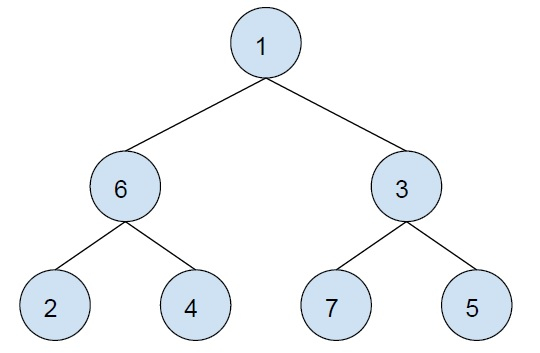# XOR of the path between any two nodes in a Binary Tree in C++

In this problem, we are given a binary tree and two nodes of the binary tree. Our task is to print the XOR of all nodes that come in the path between the two nodes.

Let’s take an example to understand the problem,We need to find the xor of all nodes between 2 and 3.

The path from 2 to 3, 2 → 6 → 1 → 3.

We will find 2^3^1^3.

Output

To solve this problem, we need to find the paths from one to another node. For this, we will find the XOR of all nodes in the path from the root to both nodes. On doing this there is two cases while traversing from the root node, either the source and destination node both lie on the same side of the root node or they lie on different sides of the root node. In the first case, all nodes that do not come in the path will be traversed twice and will be canceled out. And in the former case whole path from the root to nodes is needs to be considered. At each step we will find the XOR of the node with the previous found XOR result, this will save space.

## Example

Program to show the implementation of our solution,

Live Demo

#include <bits/stdc++.h>
using namespace std;
struct Node {
int data;
Node *left, *right;
};
struct Node* getNode(int data){
struct Node* newNode = new Node;
newNode->data = data;
newNode->left = newNode->right = NULL;
return newNode;
}
void pathStoD(Node* root, unordered_map<int, int>& path, int XOR){
if (!root)
return;
path.insert(make_pair(root->data, XOR ^ root->data));
XOR ^= root->data;
if (root->left)
pathStoD(root->left, path, XOR);
if (root->right)
pathStoD(root->right, path, XOR);
}
int findPathXOR(unordered_map<int, int> path, int node1, int node2){
return path[node1] ^ path[node2];
}
int main(){
struct Node* root = getNode(1);
root->left = getNode(6);
root->left->left = getNode(2);
root->left->right = getNode(4);
root->right = getNode(3);
root->right->left = getNode(7);
root->right->right = getNode(5);
int XOR = 0;
unordered_map<int, int> mp;
int source = 2;
int destination = 3;
pathStoD(root, mp, XOR);
cout<<"The XOR of all node from "<<source<<" to "<<destination<<" of the tree is : ";
cout<<findPathXOR(mp, source, destination);
return 0;
}

## Output

The XOR of all node from 2 to 3 of the tree is : 7